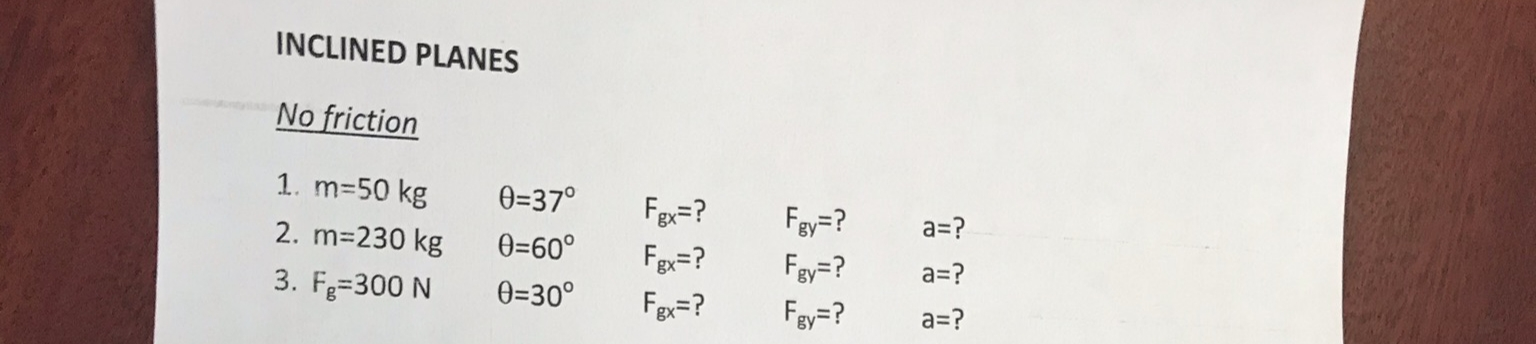# INCLINED PLANESNo friction1. m=50 kg0=37°Fgx=?Fgy=?Fgy=?a=?2. m=230 kg0=60°F=?a=?3. Fg=300 N0=30°Fgx=?Fgy=?a=?

Question
5 views

Inclined planes #2help_outlineImage TranscriptioncloseINCLINED PLANES No friction 1. m=50 kg 0=37° Fgx=? Fgy=? Fgy=? a=? 2. m=230 kg 0=60° F=? a=? 3. Fg=300 N 0=30° Fgx=? Fgy=? a=? fullscreen
check_circle

Step 1

Write the expression for the component of gravitational force along the plane

Step 2

Substitute the values

Step 3

Write the expression for the component of gravit...

### Want to see the full answer?

See Solution

#### Want to see this answer and more?

Solutions are written by subject experts who are available 24/7. Questions are typically answered within 1 hour.*

See Solution
*Response times may vary by subject and question.
Tagged in

### Science